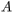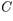# Problem: Six parallel-plate capacitors of identical plate separation have different plate areas ,different capacitances ,and different dielectrics filling the space between the plates.1. A = 4 cm2    C = 2 nF2. A = 1 cm2     C = 1 nF3. A = 2 cm2     C = 8 nF4. A = 8 cm2    C = 2 nF5. A = 4 cm2    C = 1 nF6. A = 2 cm2     C = 4 nFAll of the capacitors are now attached to batteries with the same potential difference.Rank the capacitors on the basis of the charge stored on the positive plate.Rank from largest to smallest.

###### FREE Expert Solution

The charge stored on a capacitor:

$\overline{){\mathbf{Q}}{\mathbf{=}}{\mathbf{C}}{\mathbf{V}}}$

When V is constant, the charge stored is affected by C alone.

94% (301 ratings)###### Problem Details

Six parallel-plate capacitors of identical plate separation have different plate areas,different capacitances,and different dielectrics filling the space between the plates.

1. A = 4 cm2    C = 2 nF

2. A = 1 cm2     C = 1 nF

3. A = 2 cm2     C = 8 nF

4. A = 8 cm2    C = 2 nF

5. A = 4 cm2    C = 1 nF

6. A = 2 cm2     C = 4 nF

All of the capacitors are now attached to batteries with the same potential difference.

Rank the capacitors on the basis of the charge stored on the positive plate.

Rank from largest to smallest.